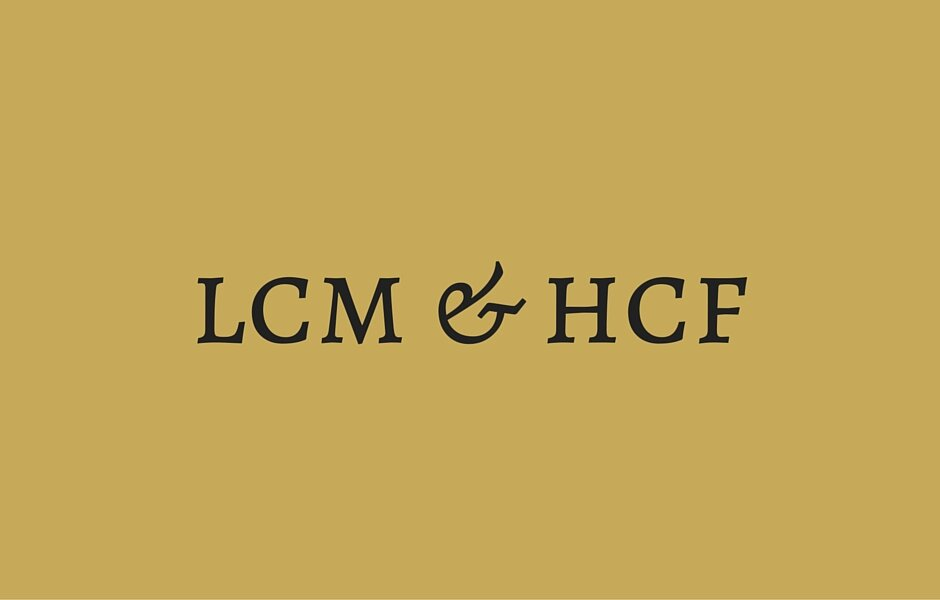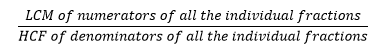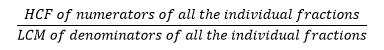Select PageOne of the most common yet neglected topics is that involving LCM (Least common multiple) and HCF (Highest common factor) or GCD (Greatest common divisor). The questions look very easy and test basic concepts but then students tend to somehow get confused and overlook certain data points probably due to shaky basics. I will be covering basics of LCM and HCF in this article along with a few questions that might be possible when it comes to CAT 2015.

Concept of LCM and HCF

LCM, as the name suggests is the lowest multiple common to two numbers. So, if you have two numbers say 4 and 6, it simply means that the first number which appears in the table of both 4 and 6 will be the LCM.

So, if we write the tables of the two numbers we get:

4, 8, 12, 16, 20, 24, 28…

6, 12, 18, 24, 30, 36, 42…

As we can see, the lowest number that is common to the two series is 12.

There is a mathematical way to do it as well. We need to simply factorize the two numbers first, then write down the common factors once and then all the remaining factors as it is and finally multiply all the numbers that you have got.

So, for example if we have to find the LCM of 12 and 39 we would write it as:

12 = 2*2*3

39 = 3*13

The common factor is 3 in this case so we take one 3 outside.

The remaining factors are 2, 2, 13 and so, we will write them down as well.

Now LCM is nothing but the product of these terms ie. 3*2*2*13 = 156

Remember that LCM of a group of numbers is always greater than or equal to the largest number.

HCF is essentially the highest common factor between can be calculated by factorizing all the individual integers and then finding out all the common factors. So, if you have two numbers say 4 and 6, it simply means that the factor that is common to both these numbers will be the HCF.

4 = 2*2

6 = 2*3

As 2 is the only factor common to both the numbers, we understand that the HCF is 2.

Similarly, if you have to find the HCF of 2990 and 13780, we factorize the two numbers as follows:

2990 = 2*5*13*23

13780 = 2*2*5*13*53

As can be seen, 2, 5 and 13 are the common factors and so, the highest common factor will be 2*5*13=130.

Remember that HCF of a group of numbers is always lesser than or equal to the smallest number.

LCM and HCF of more than two numbers

It becomes slightly tricky in cases involving more than two numbers. There are a lot of applications of this concept, particularly in time, speed and distance questions involving circular motion, derangements and. It is simply an extension of what we do in case of two numbers and so, not very difficult. Let’s go through an example:

What is the LCM of 24, 36 and 42?

We factorize the terms at first.

24 = 2*2*2*3

36 = 2*2*3*3

42 = 2*3*7

Then we write down the highest number of times a factor has appeared in the expression:

2: maximum of 3 times in 24

3: maximum of 2 times in 36

7: maximum of 1 time in 42

Multiply the above: 2*2*2*3*3*7=504

A common mistake done here is that students tend to forget about considering a factor common to two terms and end up counting it twice.

Similarly, HCF in the above case would be 6.

Special properties of LCM and HCF

• Whenever the HCF of a set of numbers (a, b, c, d,…) is known to be say some number x, these numbers can be written as ax, bx, cx, dx,… where a, b, c, d,… do not share any other factor except 1. Remember that we are talking about the entire set (a, b, c, d,…) and not individual pairs.
• Co-prime numbers: Two numbers that do not share any other factor except 1 are said to be co-prime. The LCM of such numbers is equal to the product of those numbers and the HCF is equal to 1.
• If two or more numbers leave the same remainder when divided by the same number (n), the difference between two such numbers will be completely divisible by n.
• An application can be seen in the Chinese remainder theorem wherein, if the remainders of the same number divided by different divisors are known, we can equate the two to find the remainder of the same number when divided by the LCM of the individual divisors.
• LCM of fractions can be found by using the formula:• HCF of fractions can be found by using the formula:• For any two numbers, LCM * HCF = Product of two numbers. Remember that it is applicable to two numbers only

A commonly confused scenario occurs when one is not sure whether to use LCM or HCF while solving a particular question. The thing to remember would be to understand that if you are distributing objects among n individuals such that each of them get a particular, equal quantity x, then this quantity x is the HCF of the number of individual items. Let’s check out a few examples and understand how to go about such questions.

What is the smallest number which when divided by 6, 15, 27 leaves a remainder of 5, 14 and 26 respectively?

The number can essentially be represented by:

N=6a+5=15b+14=27c+26

Using the concept of negative remainders, we can say that

N=6p-1=15q-1=27r-1

Or

N+1=6p=15q=27r

So, we know that N+1 is the smallest such number that is divisible by 6, 15 and 27. In other words, we need to find the LCM of 6, 15 and 27 and subtract 1 from it to get the value of N which would be (3*2*5*3*3) = 270-1=269.

The largest 8 digit number that would be divisible by 18, 24, 36, and 75 is?

Again, we have to take the LCM of the four terms which would give us the lowest number that would be divisible by each of 18, 24, 36 and 75. This can be found out to be = 3*6*8*12*25=43200.

Now, one way is to take multiples of 43200 and keep on trying it until we hit a number just less than 109. But that would be a bit lengthy and calculation intensive. What you can do is find the remainder of 109 mod 43200 and you should be done.

107 mod 432

54 * 103 mod 27

625000 mod 27

4

Multiplying back by the cancelled factors, we get 4*16*100=6400.

So, we simply subtract 6400 from 108 and arrive at the answer to be 99993600.

Three bells chime at an interval of 18 min, 24 min and 32 min. At a certain time they begin to chime together. What length of time will elapse before they chime together again? (CAT 1995)

(a) 2 hours and 24 minutes

(b) 4 hours and 48 minutes

(c) 1 hour and 36 minutes

(d) 5 hours

Simply put, we have to understand the LCM of 18, 24, 32 and that would be our answer. This because, the first bell will chime at times 18 min, 36 min, 54 min and so on. The second bell will chime at times 24 min, 48 min, 72 min and so on. The third bell will chime at times 32 min, 64 min, 96 min and so on.

So, LCM of 18, 24, and 32 is given by: 25 * 32 = 288 minutes and so, option (b) is correct.

What is the number x? (CAT 1995)

1. The LCM of x and 18 is 36

2. The HCF of x and 18 is 2

Using statement 1, we get that x could be either 4, 12 or 36 and so, it cannot be uniquely determined

Using statement 2, we cannot get a single value of x and so, it is not sufficient

Using both the statements, we can apply the rule of product of two numbers being equal to the products of their LCM and HCF and get a unique value of x.

A few more questions from previous year CAT papers that would be good for practicing:

A is the set of positive integers such that when divided by 2, 3, 4, 5, 6 leaves the remainders 1, 2, 3, 4, 5 respectively. How many integers between 0 and 100 belong to set A? (CAT 1998)

(a) 0             (b) 1             (c) 2             (d) None of these

Number of students who have opted for subjects A, B and C are 60, 84 and 108 respectively. The examination is to be conducted for these students such that only the students of the same subject are allowed in one room. Also the number of students in each room must be same. What is the minimum number of rooms that should be arranged to meet all these conditions?

(a) 28           (b) 60           (c) 12           (d) 21

For two positive integers a and b define the function h(a,b) as the greatest common factor (G.C.F) of a, b. Let A be a set of n positive integers. G(A), the G.C.F of the elements of set A is computed by repeatedly using the function h. The minimum number of times h is required to be used to compute G is (CAT 1999)

(a) n/2         (b) (n – 1)     (c) n             (d) None of these

The integers 34041 and 32506, when divided by a three-digit integer n, leave the same remainder. What is the value of n? (CAT 2000)

(a) 289         (b) 367         (c) 453         (d) 307

A red light flashes three times per minute and a green light flashes five times in 2 minutes at regular intervals. If both lights start flashing at the same time, how many times do they flash together in each hour?

(a) 30           (b) 24           (c) 20           (d) 60

Of 128 boxes of oranges, each box contains at least 120 and at most 144 oranges. The number of boxes containing the same number of oranges is at least

(a) 5             (b) 103         (c) 6             (d) Cannot be determined

error: Content is protected !!